Next: Heat engines Up: Classical thermodynamics Previous: The isothermal atmosphere

Of course, we know that the atmosphere is not isothermal. In fact, air temperature falls quite noticeably with increasing altitude. In ski resorts, you are told to expect the temperature to drop by about 1 degree per 100 meters you go upwards. Many people cannot understand why the atmosphere gets colder the higher up you go. They reason that as higher altitudes are closer to the Sun they ought to be hotter. In fact, the explanation is quite simple. It depends on three important properties of air. The first important property is that air is transparent to most, but by no means all, of the electromagnetic spectrum. In particular, most infrared radiation, which carries heat energy, passes straight through the lower atmosphere and heats the ground. In other words, the lower atmosphere is heated from below, not from above. The second important property of air is that it is constantly in motion. In fact, the lower 20 kilometers of the atmosphere (the so called troposphere) are fairly thoroughly mixed. You might think that this would imply that the atmosphere is isothermal. However, this is not the case because of the final important properly of air: i.e., it is a very poor conductor of heat. This, of course, is why woolly sweaters work: they trap a layer of air close to the body, and because air is such a poor conductor of heat you stay warm.

Imagine a packet of air which is being swirled around in the atmosphere. We would expect it to always remain at the same pressure as its surroundings, otherwise it would be mechanically unstable. It is also plausible that the packet moves around too quickly to effectively exchange heat with its surroundings, since air is very a poor heat conductor, and heat flow is consequently quite a slow process. So, to a first approximation, the air in the packet is adiabatic. In a steady-state atmosphere, we expect that as the packet moves upwards, expands due to the reduced pressure, and cools adiabatically, its temperature always remains the same as that of its immediate surroundings. This means that we can use the adiabatic gas law to characterize the cooling of the atmosphere with increasing altitude. In this particular case, the most useful manifestation of the adiabatic law is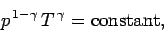(329)

giving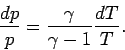(330)

Combining the above relation with the equation of hydrostatic equilibrium, (326), we obtain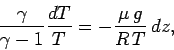(331)

or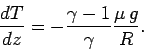(332)

Now, the ratio of specific heats for air (which is effectively a diatomic gas) is about 1.4 (see Tab. 2). Hence, we can calculate, from the above expression, that the temperature of the atmosphere decreases with increasing height at a constant rate ofcentigrade per kilometer. This value is called the adiabatic lapse rate of the atmosphere. Our calculation accords well with the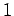degree colder per 100 meters higher'' rule of thumb used in ski resorts. The basic reason why air is colder at higher altitudes is that it expands as its pressure decreases with height. It, therefore, does work on its environment, without absorbing any heat (because of its low thermal conductivity), so its internal energy, and, hence, its temperature decreases.

According to the adiabatic lapse rate calculated above, the air temperature at the cruising altitude of airliners (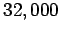feet) should be about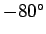centigrade (assuming a sea level temperature ofcentigrade). In fact, this is somewhat of an underestimate. A more realistic value is about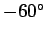centigrade. The explanation for this discrepancy is the presence of water vapour in the atmosphere. As air rises, expands, and cools, water vapour condenses out releasing latent heat which prevents the temperature from falling as rapidly with height as the adiabatic lapse rate would indicate. In fact, in the Tropics, where the humidity is very high, the lapse rate of the atmosphere (i.e., the rate of decrease of temperature with altitude) is significantly less than the adiabatic value. The adiabatic lapse rate is only observed when the humidity is low. This is the case in deserts, in the Arctic (where water vapour is frozen out of the atmosphere), and, of course, in ski resorts.

Suppose that the lapse rate of the atmosphere differs from the adiabatic value. Let us ignore the complication of water vapour and assume that the atmosphere is dry. Consider a packet of air which moves slightly upwards from its equilibrium height. The temperature of the packet will decrease with altitude according to the adiabatic lapse rate, because its expansion is adiabatic. We assume that the packet always maintains pressure balance with its surroundings. It follows that since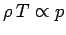, according to the ideal gas law, then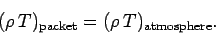(333)

If the atmospheric lapse rate is less than the adiabatic value then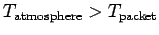implying that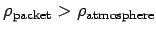. So, the packet will be denser than its immediate surroundings, and will, therefore, tend to fall back to its original height. Clearly, an atmosphere whose lapse rate is less than the adiabatic value is stable. On the other hand, if the atmospheric lapse rate exceeds the adiabatic value then, after rising a little way, the packet will be less dense than its immediate surroundings, and will, therefore, continue to rise due to buoyancy effects. Clearly, an atmosphere whose lapse rate is greater than the adiabatic value is unstable. This effect is of great importance in Meteorology. The normal stable state of the atmosphere is for the lapse rate to be slightly less than the adiabatic value. Occasionally, however, the lapse rate exceeds the adiabatic value, and this is always associated with extremely disturbed weather patterns.

Let us consider the temperature, pressure, and density profiles in an adiabatic atmosphere. We can directly integrate Eq. (332) to give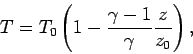(334)

where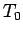is the ground level temperature, and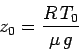(335)

is the isothermal scale-height calculated using this temperature. The pressure profile is easily calculated from the adiabatic gas law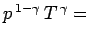constant, or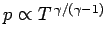. It follows that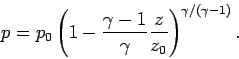(336)

Consider the limit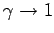. In this limit, Eq. (334) yields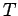independent of height (i.e., the atmosphere becomes isothermal). We can evaluate Eq. (336) in the limit asusing the mathematical identity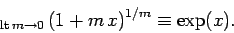(337)

We obtain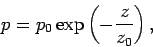(338)

which, not surprisingly, is the predicted pressure variation in an isothermal atmosphere. In reality, the ratio of specific heats of the atmosphere is not unity, it is about 1.4 (i.e., the ratio for diatomic gases), which implies that in the real atmosphere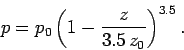(339)

In fact, this formula gives very similar results to the exponential formula, Eq. (338), for heights below one scale-height (i.e.,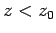). For heights above one scale-height, the exponential formula tends to predict too low a pressure. So, in an adiabatic atmosphere, the pressure falls off less quickly with altitude than in an isothermal atmosphere, but this effect is only really noticeable at pressures significantly below one atmosphere. In fact, the isothermal formula is a pretty good approximation below altitudes of about 10 kilometers. Since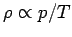, the variation of density with height goes like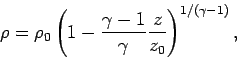(340)

where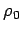is the density at ground level. Thus, the density falls off more rapidly with altitude than the temperature, but less rapidly than the pressure.

Note that an adiabatic atmosphere has a sharp upper boundary. Above height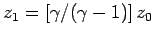the temperature, pressure, and density are all zero: i.e., there is no atmosphere. For real air, with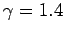,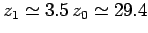kilometers. This behaviour is quite different to that of an isothermal atmosphere, which has a diffuse upper boundary. In reality, there is no sharp upper boundary to the atmosphere. The adiabatic gas law does not apply above about 20 kilometers (i.e., in the stratosphere) because at these altitudes the air is no longer strongly mixed. Thus, in the stratosphere the pressure falls off exponentially with increasing height.

In conclusion, we have demonstrated that the temperature of the lower atmosphere should fall off approximately linearly with increasing height above ground level, whilst the pressure should fall off far more rapidly than this, and the density should fall off at some intermediate rate. We have also shown that the lapse rate of the temperature should be about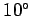centigrade per kilometer in dry air, but somewhat less than this in wet air. In fact, all off these predictions are, more or less, correct. It is amazing that such accurate predictions can be obtained from the two simple laws,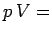constant for an isothermal gas, and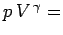constant for an adiabatic gas.Next: Heat engines Up: Classical thermodynamics Previous: The isothermal atmosphere
Richard Fitzpatrick 2006-02-02